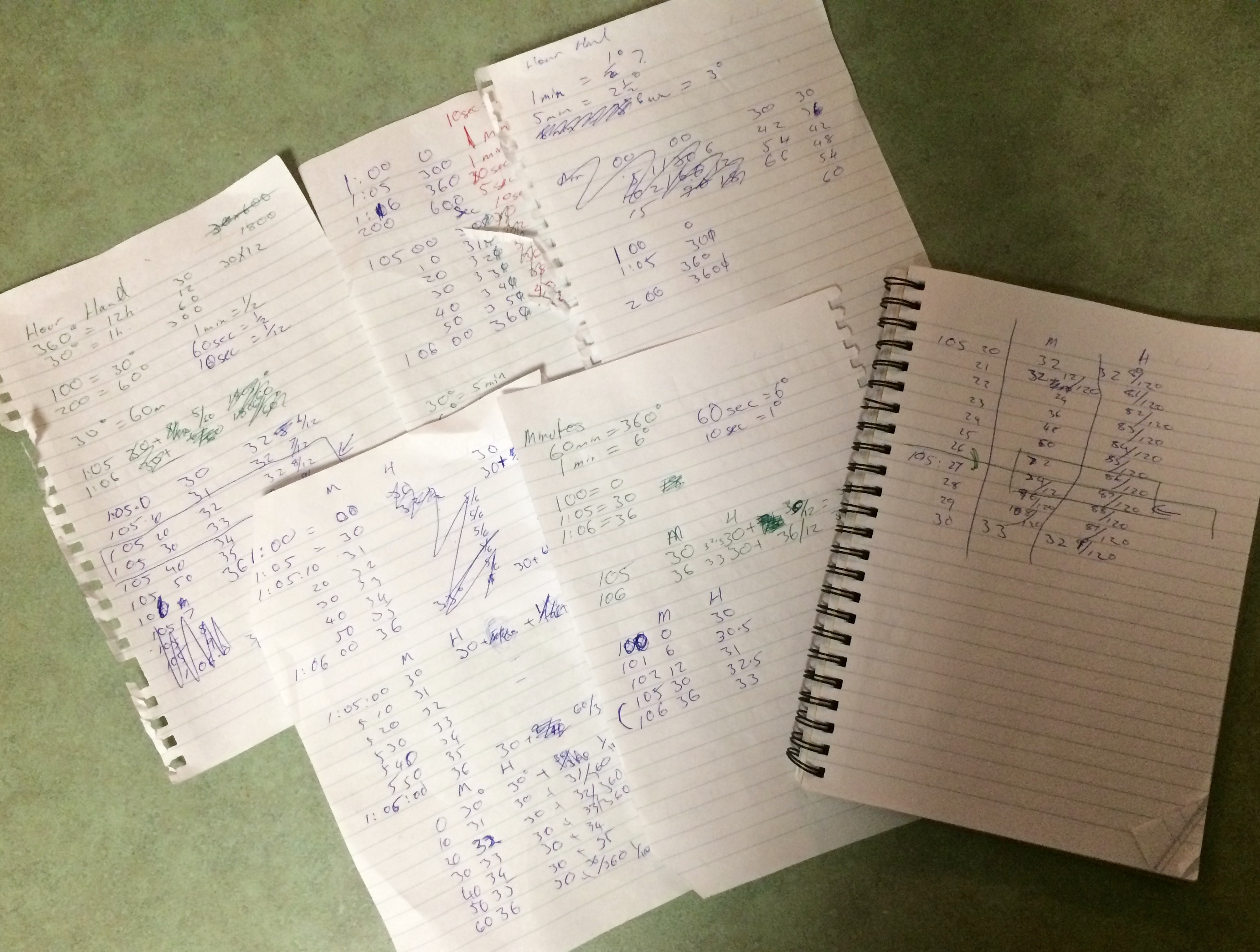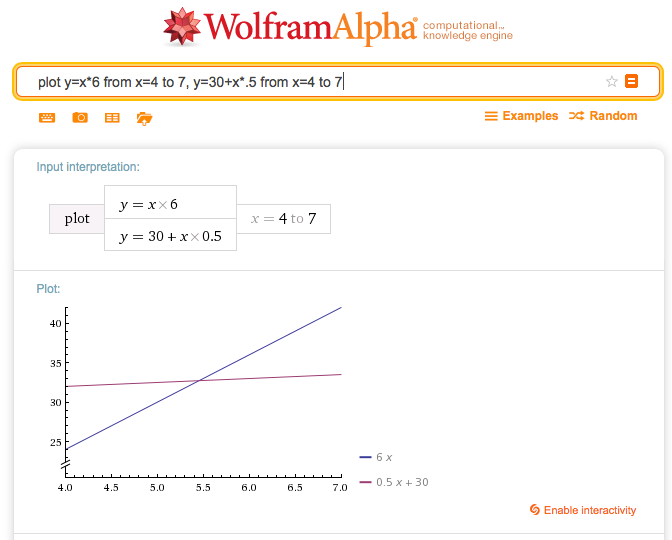# Homework Help: Time Passing

I was asked to help with some math homework tonight, and the question was as follows:

Between 1pm and 2pm the minute hand will cross the hour hand, at what time will they cross?

# Working it outA basic understanding of a clock means that 1 o’clock and 5 minutes past the hour share the same spot, meaning that’s the earliest they can possibly cross, that’s a good starting point. Thinking about it means they won’t cross precisely on that point, but it’ll happen very soon after.

Going back to the most basic principles we get:

• Clocks are round, and the hands rotate 360 degrees around a central point.
• The hour hand shows 12 hours per revolution,
• The minute hand shows sixty minutes per revolution.

This means that with every minute that passes,

• the hour hand moves 12 a degree,
• the minute hand moves 6 degrees

I’m doing this entirely by hand so that I understand it better and can explain it to someone else. After many, many pieces of paper wasted with distracting failures of logic I was able to calculate the answer down to a 1 second range and explain how I got there!

There’s three iterations in this explanation, and each table has time, minute hand angle and hour hand angle. I’m working in fractions because decimal’s nutty for this kind of problem.

## First Iteration

To break the problem down into minutes, I drew a table to check my assumptions.

Time Minute Hand Hour Hand
1:00 30
1:01 6 30 1/2
1:02 12 31
1:03 18 31 1/2
1:04 24 32
1:05 30 32 1/2
1:06 36 33
1:07 42 33 1/2
.. ..
2:00 60

Once the minute hand’s angle has increased past the hour hand’s angle, we’ve satisfied our requirement, so they cross over at somewhere between 1:05 and 1:06.

## Second Iteration

Next up, I broke the time into 10-second blocks. Each ten seconds is a degree for the minute hand, and 112 of a degree for the hour hand.

Time Minute Hand Hour Hand
1:05:00 30 32 6/12
1:05:10 31 32 7/12
1:05:20 32 32 8/12
1:05:30 33 32 9/12
1:05:40 34 32 10/12
1:05:50 35 32 11/12
1:06:00 36 33

Getting closer again, this time they cross between 1:05:20 and 1:05:30. Somewhere between 32 812 and 32 912 degrees.

## Third Iteration

This next table breaks it down into seconds. The fractions might look completely loopy here, but I did it to make comparison easy. 1 second is 0.1 degrees on the minute hand, but it’s 1120 of a degree on the hour hand so I moved the minute hand to 12120 per tick.

Time Minute Hand Hour Hand
1:05:20 32 0/120 32 80/120
1:05:21 32 12/120 32 81/120
1:05:22 32 24/120 32 82/120
1:05:23 32 36/120 32 83/120
1:05:24 32 48/120 32 84/120
1:05:25 32 60/120 32 85/120
1:05:26 32 72/120 32 86/120
1:05:27 32 84/120 32 87/120
1:05:28 32 96/120 32 88/120
1:05:29 32 108/120 32 89/120
1:05:30 32 120/120 (33) 32 90/120

1:05:27. That’s good enough for me!

# Graphing it

A way to find the problem visually (without a working clock) is to graph the movement of the hands.

Minute hand angle is simple, being a function of

``````angle(minutes) = minutes * 6
``````

Hour hand angle’s a little different, being a function of both the hour and the minutes.

``````angle(hours, minutes) = ( 30 * hours ) + ( minutes * 0.5 )
``````

To graph this particular example in a spreadsheet program we could make a table of minutes and do some trickery, but I realised I could use Wolfram Alpha to calculate it magically (It was that, or stuff around with matplotlib etc). After five minutes of clicking around in the site I was able to ask it:

plot y=x6 from x=4 to 7, y=30+x.5 from x=4 to 7

Limiting the graph made it a little easier to see, and it showed precisely what I wanted to get to, the cross over point of the two hands!There’s a few different ways to do this, which I searched for after I had solved it on paper. A Mathematica StackExchange Thread has some great ways of working it out in that program and visualising it.

# Angle calculation table

I ended up writing this out in a variety of different forms to check my assumptions and help my feeble brain to be able to store the comparisons.

## Hour hand

• 60 minutes = 30 degrees
• 5 minutes = 2 12 degrees
• 1 minute = 12 degree
• 60 seconds = 12 degree
• 10 seconds = 112 degree

## Minute hand

• 60 minutes = 360 degrees
• 6 minutes = 36 degrees
• 1 minute = 6 degrees
• 60 seconds = 6 degrees
• 10 seconds = 1 degree
• 1 second = 0.1 degrees